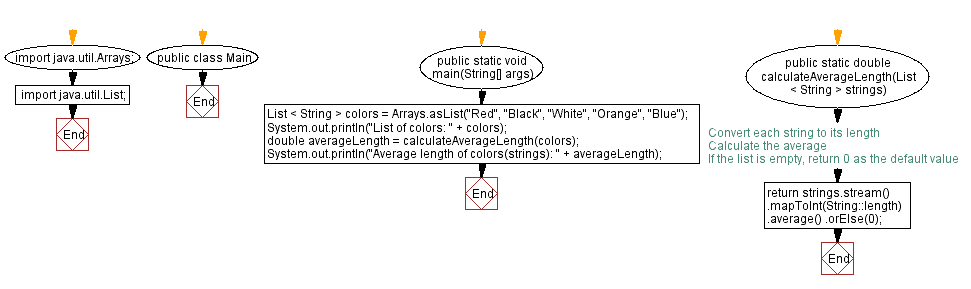# Java Program: Lambda expression to find average string length

## Java Lambda Program: Exercise-23 with Solution

Write a Java program to implement a lambda expression to find the average length of strings in a list.

Sample Solution:

Java Code:

``````import java.util.Arrays;
import java.util.List;

public class Main {
public static void main(String[] args) {
List <String> colors = Arrays.asList("Red", "Black", "White", "Orange", "Blue");
System.out.println("List of colors: " + colors);

double averageLength = calculateAverageLength(colors);
System.out.println("Average length of colors(strings): " + averageLength);
}

public static double calculateAverageLength(List < String > strings) {
return strings.stream()
.mapToInt(String::length) // Convert each string to its length
.average() // Calculate the average
.orElse(0); // If the list is empty, return 0 as the default value
}
}
``````

Sample Output:

```List of colors: [Red, Black, White, Orange, Blue]
Average length of colors(strings): 4.6
```

Explanation:

In the above exercise,

The main() method creates a list of strings (strings) containing four elements.

Following are the steps taken by the calculateAverageLength method when given a list of strings:

• The stream() method is used on the strings list to create a stream of strings.
• The mapToInt method converts each string to its length by using a method reference String::length.
• The average method calculates the average length of the strings in the stream.
• The orElse method is used to provide a default value of 0 if the list is empty.

The calculated average length is returned by the calculateAverageLength method and stored in the averageLength variable.

Flowchart:Live Demo:

Java Code Editor:

Improve this sample solution and post your code through Disqus

Java Lambda Exercises Previous: Lambda expression for checking uppercase, lowercase, or mixedcase strings.
Java Lambda Exercises Next: Lambda expression to find largest prime factor.

What is the difficulty level of this exercise?

Test your Programming skills with w3resource's quiz.

﻿

## Java: Tips of the Day

How do I remove repeated elements from ArrayList?

If you don't want duplicates in a Collection, you should consider why you're using a Collection that allows duplicates. The easiest way to remove repeated elements is to add the contents to a Set (which will not allow duplicates) and then add the Set back to the ArrayList:

```Set<String> set = new HashSet<>(yourList);
yourList.clear();